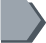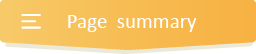Your browser does not support JavaScript!

#Comparing and ordering word problems 2nd grade### What are some basic steps to consider when comparing and ordering numbers word problems?

It is only in this great resource that you will discover what are some basic steps to consider when comparing are and ordering numbers word problems? To compare and order numbers, therefore,

First of all, count the number of digits each number has. The number with more digits means it’s the greatest. But if all the numbers have the same number of digits, then you’ll need to compare the digits in the following place values to the right.

Keep comparing from one place value to the next until you find the number with a higher particular place value than the other. That number will thus be the larger one.

•